# Long Straddle Payoff, Risk and Break-Even Points

This page explains long straddle profit and loss at expiration and the calculation of its risk and break-even points.

Long straddle is a position consisting of a long call option and a long put option, both with the same strike and the same expiration date.

It is a non-directional long volatility strategy. It is generally suitable when you expect the underlying security to be very volatile and move a lot, but you are not sure whether the price move will be up or down. The position makes a profit when your expectation is correct and the underlying does make a big move to one or the other side. If you are wrong and the underlying price stays more or less the same, the trade makes a loss.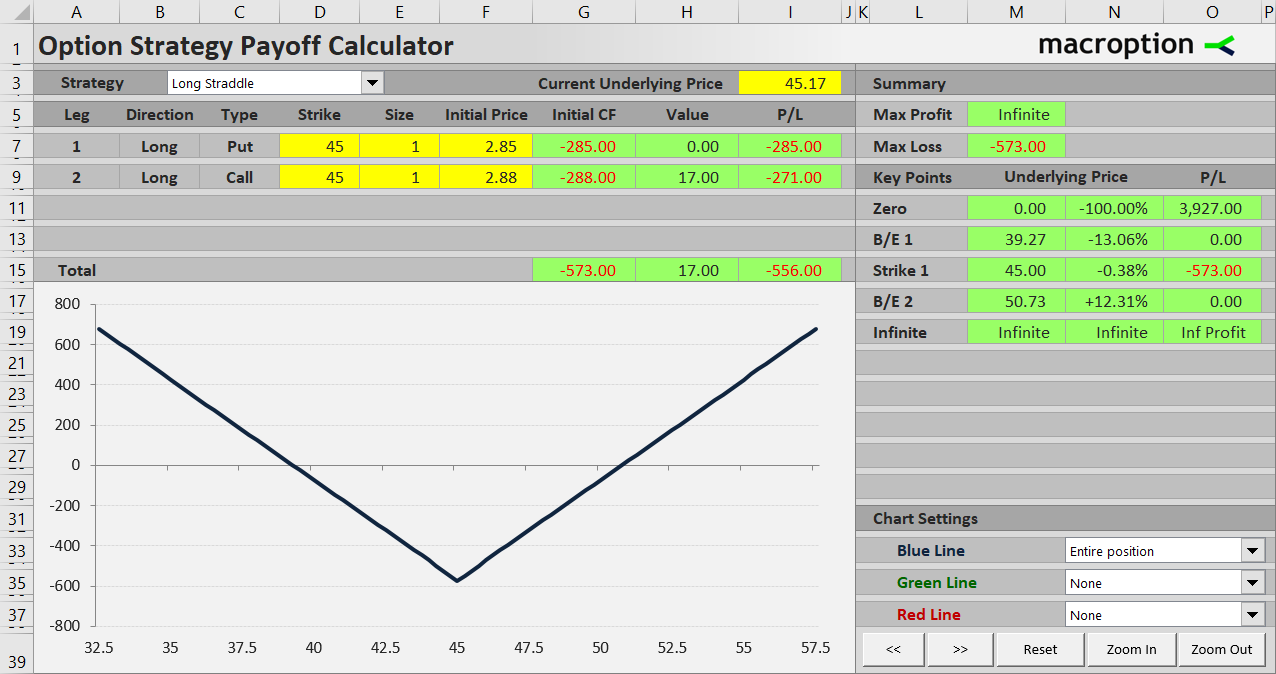Long straddle has limited risk, equal to the premium paid for both legs, and unlimited potential profit.

Let’s explain the payoff on an example, and have a look at the sources of its risk and profit exposures.

Consider a straddle created with the following two transactions:

• Buy a \$45 strike put option for \$2.85 per share.
• Buy a \$45 strike call option with the same expiration date for \$2.88 per share.

The underlying security is trading somewhere close to \$45 at the moment. Typically, at the money options – the strike nearest to the current underlying price – are selected. This helps balance directional exposure of the two legs and make the position non-directional overall, so the trader can focus on volatility, which is really the main idea of a straddle (in some cases, a trader may choose a different strike to intentionally create a straddle with a little directional bias; this is more advanced and we will not consider it for now).

## Initial Cost

Initial cost of the position is very easy to calculate: just add up the money paid for the two legs.

Initial cost = put cost + call cost

In our example:

Initial cost = \$2.85 + \$2.88 = \$5.73 per share = \$573 per contract

(assuming standard US equity option contracts, which represent 100 shares)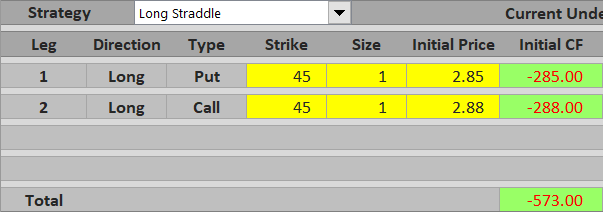## Payoff and Profit Drivers

Because the call and the put have the same strike price (\$45 in our example), only one of them is in the money at any time. When underlying price is above the strike, the call is in the money and the put is out of the money. Below the strike it’s the opposite.

Therefore, at expiration when no time value is left, one of the options can almost always (unless underlying price end up exactly at the strike) be exercised for some gain (which may or may not be enough to cover the initial cost) and the other option expires worthless.

You can see that in the payoff diagram below, which shows the straddle from our example, including the long call (green) and the long put (red).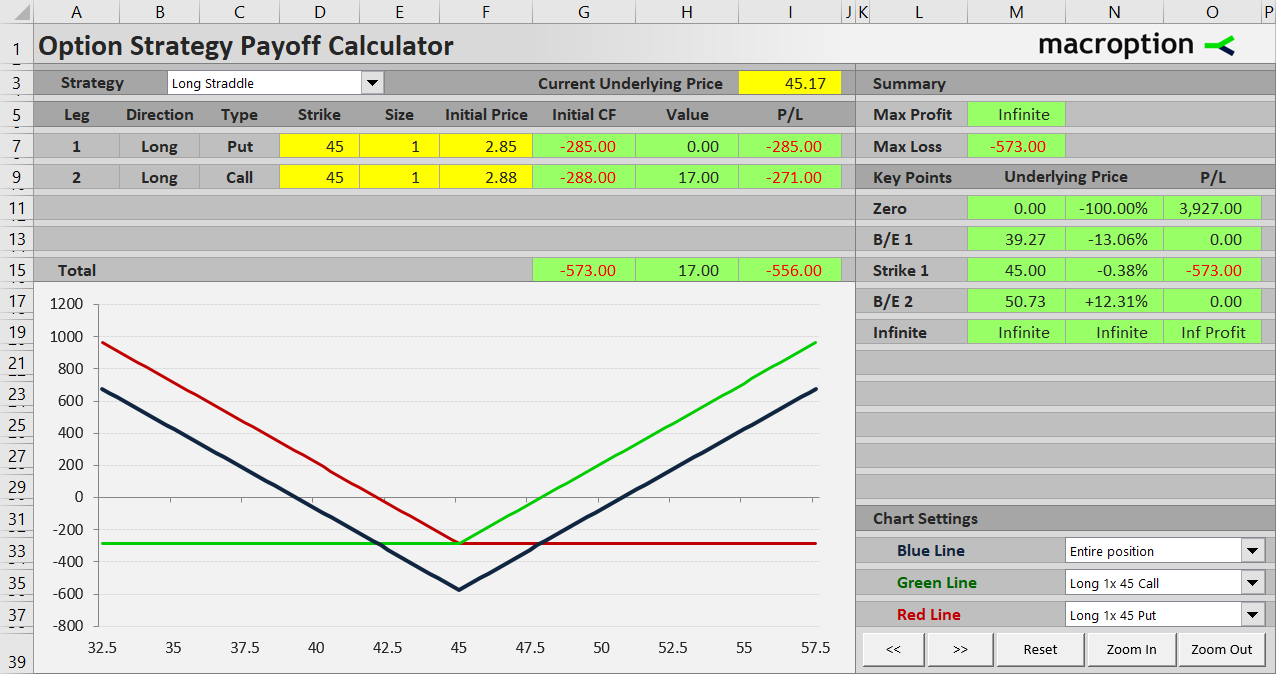## Maximum Loss (Risk)

As you can see in the payoff diagram, total P/L reaches its minimum when underlying price is exactly at the strike. This is the only point where both the call and the put have zero value at expiration. Therefore, it is exactly at the strike where the trade’s overall P/L equals maximum loss, which equals initial cost.

Maximum loss = initial cost

Regardless of what happens in the market, you can’t lose more than what you have paid for the options, because the position only includes long options (no shorts) and there is no risk of assignment or negative cash flow at expiration.

When underlying price moves away from the strike to one side or the other, increasing value of the in the money option starts to reduce the loss and, if the underlying moves far enough, eventually turns the trade into a profit. Let’s see a few example scenarios.

## When Underlying Price Goes Up

If underlying price ends up at \$49 at expiration, or \$4 above the strike, the call option is in the money and makes \$4 per share, or \$400 for one contract. The put is out of the money and expires worthless. The \$400 is not enough to cover the initial cost of both options, therefore the trade ends in a loss (\$400 – \$573 = – \$173), although much smaller than the maximum loss possible (\$573).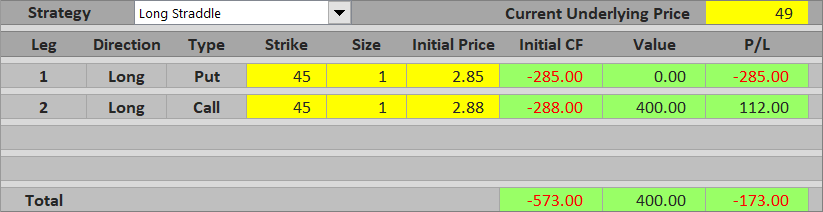If underlying price gets further up to \$54, the call is worth \$9 per share, or \$900 per contract and the trade makes a profit (\$900 – \$573 = \$327).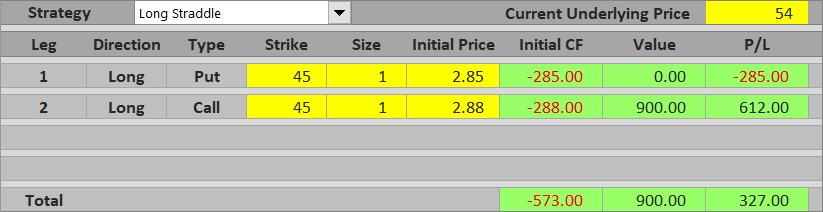## When Underlying Price Goes Down

Below the strike it works in the same way, only the put is in the money and drives the profitability, while the call expires worthless.

If underlying price is at \$43, the put is worth \$200 and overall P/L is – \$373, still a loss.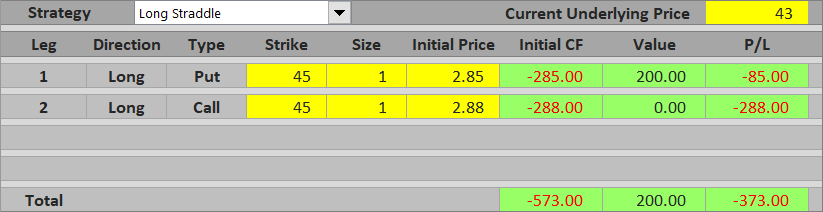If the underlying falls to \$37, the straddle makes a profit of \$227.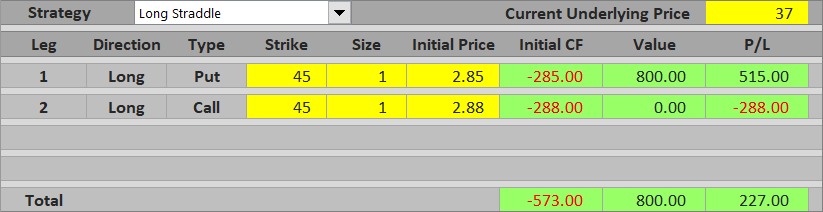Where exactly are the points where the straddle starts being profitable. How far does the underlying need to move? It is very easy to calculate.

A straddle has two break-even points.

The lower break-even point is the underlying price at which the put option’s value equals initial cost of both options.

B/E #1 = strike – initial cost

In our example:

B/E #1 = \$45 – \$5.73 = \$39.27

The upper break-even point is where the call option’s value equals initial cost of both option.

B/E #2 = strike + initial cost

In our example:

B/E #2 = \$45 + \$5.73 = \$50.73

As you can see, the underlying in our example needs to make a fairly big move for the trade to make a profit (12.7% to either side, assuming it is exactly at \$45 when opening the position). High initial cost and often very wide window of loss is one disadvantage of long straddles (and many other long volatility strategies) – while you have the luxury of not having to worry about the direction, you pay for it in the high cost of the position. Therefore, the difficulty and risk of long straddles must not be underestimated. While it looks attractive and safe when looking at the payoff diagrams, it is not that easy to trade straddles profitably in practice.

## Similar Option Strategies

Another strategy very similar to long straddle in virtually all characteristics (non-directional, long volatility, limited risk, unlimited upside) is long strangle. The main difference is that in a strangle the call and the put have different strikes. As a result, initial cost and maximum loss is lower, but the size of the move needed to reach break-even is even further, due to the distance between strikes.

The other side of a long straddle trade is short straddle – a non-directional, short volatility strategy with limited profit and unlimited risk. Similarly, the inverse of long strangle is short strangle.

Have a question or feedback? It takes less than a minute.